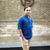# Classification using Raster Calculator

174
2
06-14-2021 12:43 AMNew Contributor

Hello,

I am trying to classify an image using raster calculator and conditional function, however, I can't seem to classify it. Can anyone kindly suggest what went wrong? Ps. The code is attached below (Int_tif2 is my image's name):

Con("Int_tif2"<= 110,0,"Int_tif2"),Con(("Int_tif2"> 110) &("Int_tif2" <= 250),1,"Int_tif2" ),Con(("Int_tif2">250) & ("Int_tif2" <= 350),2,"Int_tif2"), Con("Int_tif2" > 350,3,"Int_tif2")

Thank you

2 RepliesbyMVP Esteemed Contributor
``````Con("Int_tif2"<= 110,0, "Int_tif2",
Con(("Int_tif2"> 110) &("Int_tif2" <= 250), 1,
Con(("Int_tif2">250) & ("Int_tif2" <= 350), 2,
Con("Int_tif2" > 350, 3, "Int_tif2"))))``````

perhaps, but if it is < 110 or > 350 it will be classified as Int_tif2, if that is correct

... sort of retired...byMVP Esteemed Contributor

Looking to classify the raster into 04 classes, i.e. 0-3?

You can check the following expression.

``Con("Int_tif2"<= 110, 0, Con(("Int_tif2"> 110) &("Int_tif2" <= 250), 1, Con(("Int_tif2">250) & ("Int_tif2" <= 350), 2, Con("Int_tif2" > 350, 3))))``

Think Location